## Steady Temperature in a Rectangle.

Consider the steady temperature in a rectangle caused by heating on one face. Of the other faces, two are at zero temperature and one is insulated. The temperature satisfies the following equations: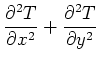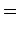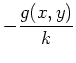(31)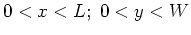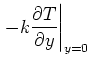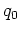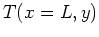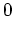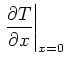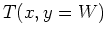This is case (X21B00)(Y21B10). The GF solution has the form: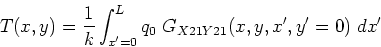(32)

There are three forms of the steady GF for the rectangle, a double-sum expression (usually to be avoided) and two single-sum expressions. Both single-sum forms of the GF form produce convergent temperature expressions, but the speed of convergence varies through the rectangle. In this example one form of the GF will be discussed, that with kernel functions along the x-axis: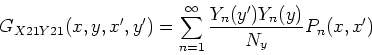(33)

The eigenfunction is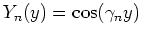, the eigenvalues are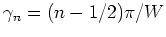and the norm is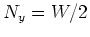. The kernel function is given by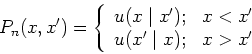(34)

where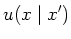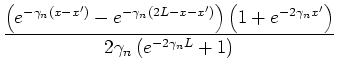Replace the GF into the temperature expression, and carry out the integral to find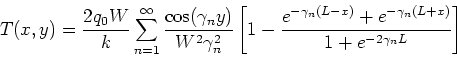(35)

A full discussion of the above integral and numerical values for the temperature are given in a paper Green's Functions, Temperature and Heat Flux in the Rectangle'' by Kevin D. Cole and David H. Yen, accepted November 2000 for the International Journal of Heat and Mass Transfer.